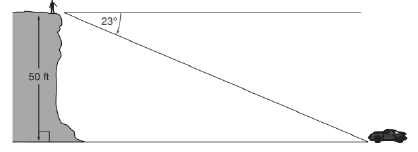Chapter 11.1, Problem 31EElementary Geometry For College St...

7th Edition
Alexander + 2 others
ISBN: 9781337614085

Solutions

Chapter
SectionElementary Geometry For College St...

7th Edition
Alexander + 2 others
ISBN: 9781337614085
Textbook Problem

In Exercises 27 to 34, use the drawings, where provided, to solve each problem. Angle measures should be given to the nearest degree; distances should be given to the nearest tenth of a unit.From a cliff, Phillip observes an automobile through an angle of depression of 23◦. If the cliff is 50ft high, how far is the automobile from Phillip?To determine

To find:

The distance between the automobile and Phillip.

Explanation

In a right angle triangle,

1) The side PQ, which is opposite to the right angle PRQ is called the hypotenuse.

(The hypotenuse is the longest side of the right triangle.)

2) The side RQ is called the adjacent side of angle θ.

3) The side PR is called the opposite side of angle θ.

Hence, the sine ratio for an acute angle is oppositehypotenuse

Given:

From, the given diagram,

Angle of depression is 23, opposite side = 50 ft(Cliff height).

Let a be the hypotenuse, that is the distance between automobile and Phillip.

We know that,

sinθ=oppositeHypotenuse

sin23°=50a

From the table 11

Still sussing out bartleby?

Check out a sample textbook solution.

See a sample solution

The Solution to Your Study Problems

Bartleby provides explanations to thousands of textbook problems written by our experts, many with advanced degrees!

Get Started

Subtract and check: 417,286287,156

Elementary Technical Mathematics

Which is the best graph of y = f (x) for the given graph of y = f (x)? a) None of these

Study Guide for Stewart's Single Variable Calculus: Early Transcendentals, 8th

True or False: converges.

Study Guide for Stewart's Multivariable Calculus, 8th